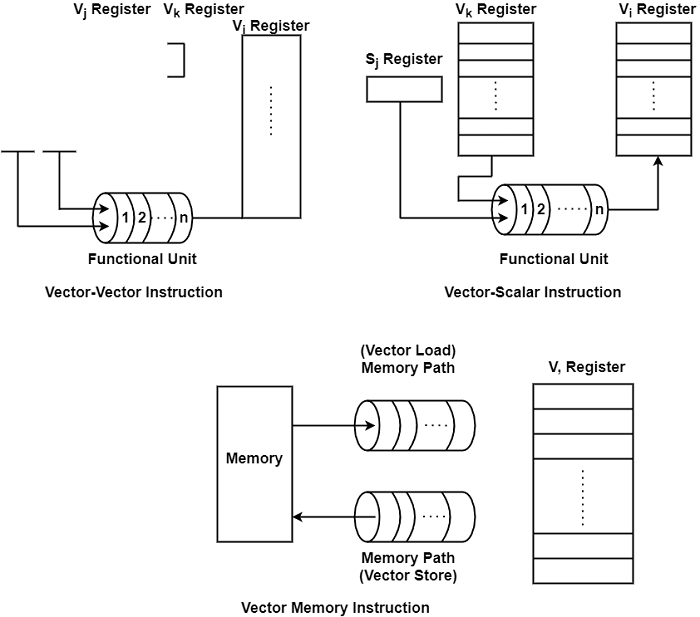# What are the types of Vector Instructions?

A vector is a set of scalar data elements, all of similar type, saved in memory. The vector elements are ordered to have a permanent addressing increment between successive elements known as the stride.

A vector processor is an ensemble of hardware resources, such as vector registers, functional pipelines, processing elements, and register counters, for executing vector operations.

Vector processing appears when arithmetic or logical operations are used to vectors. It is distinguished from scalar processing which operates on one or one pair of data. The conversion from scalar code to vector code is known as vectorization.

## Types of Vector Instructions

There are various types of vector instructions which are as follows −

• Vector-vector instructions − One or two vector operands are fetched from the specific vector registers, introduce through a functional pipeline unit and make results in another vector register. These instructions are defined by the following two mappings −

f1 : vi → vj

f2: vj x vk − vi

Example − $V\:=\sin(v_{2})$ and v3 = v1 + v2 for the mapping f1and f2 respectively, where V for i = 1,2, and 3 are vector registers.

• Vector-scalar instructions − As shown in the figure, it shows a vector-scalar instruction corresponding to the following mapping −

f3: s x vi − vj

An example is a scalar product s x v1 = v2 in which the elements of V are each multiplied by a scalar s to produce vector v2 of equal length.

• Vector-memory instructions − This corresponds to vector load or vector store element by element, between the vector register (V) and the memory (M) as defined below −

f4: M → V Vector Load

f5: V → M Vector Store• Vector reduction instructions − These correspond to the following mappings −

f6: vi − sj

f7: v x vj − sk

• Gather and scatter instructions − These instructions use two vector registers to gather or to scatter vector elements randomly throughout the memory, corresponding to the following mappings −

a: M → v1 x v0 Gather

fa: v1 x v0 − M Scatter

Gather is an operation that fetches from memory the nonzero elements of a sparse vector using indices that themselves are indexed.

Scatter does the opposite, storing into memory a vector in a sparse vector whose nonzero entries are indexed. The vector register contains the data and the vector register is used as an index to gather or scatter data from or to random memory locations respectively.

• Masking instructions − In masking instructions, it uses a mask vector to wrap or to broaden a vector to a shorter or longer index vector, respectively, equivalent to the following mappings −

f10: v0x vm → v1